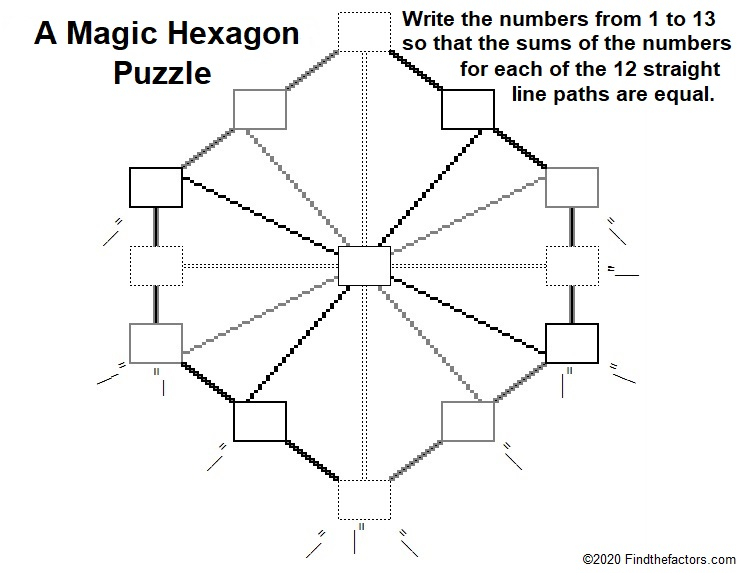# 1569 A Magic Hexagon Puzzle

Contents

### Today’s Puzzle:

The hexagon below has six edges and six lines going through its center. Can you write the numbers from 1 to 13 in the boxes so that the sums of the numbers along each of those lines are equal? The solution can be written 12 different ways, but they are all rotations and/or reflections of each other.It should be helpful to realize that
1 + 13 = 14,
2 + 12 = 14,
3 + 11 = 14,
4 + 10 = 14,
5 + 9 = 14, and
6 + 8 = 14.
That leaves a 7 without a partner. Where do you think the 7 should go? What do you think the magic sum of each of the twelve paths will be?

Should even numbers or odd numbers go in the corners of the hexagon?

### Factors of 1569:

This is my 1569th post. What are the factors of 1569?

• 1569 is a composite number.
• Prime factorization: 1569 = 3 × 523.
• 1569 has no exponents greater than 1 in its prime factorization, so √1569 cannot be simplified.
• The exponents in the prime factorization are 1 and 1. Adding one to each exponent and multiplying we get (1 + 1)(1 + 1) = 2 × 2 = 4. Therefore 1569 has exactly 4 factors.
• The factors of 1569 are outlined with their factor pair partners in the graphic below.### More about the Number 1569:

1569 is the difference of two squares:
263² – 260².

1569 is also the sum of the six numbers from 259 to 264.

Do you see any relationship between those two facts?

Since 1569 is divisible by 3, it is the magic sum of the magic hexagon shown below:This site uses Akismet to reduce spam. Learn how your comment data is processed.